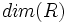# Krull dimension

## Definition

### Symbol-free definition

The Krull dimension of a commutative unital ring is the supremum of lengths of descending chains of distinct prime ideals.

### Definition with symbols

Let$R$ be a commutative unital ring. The Krull dimension of$R$, denoted$dim(R)$ is the supremum over all$n$ for which there exist strictly descending chains of prime ideals:$P_0 \supset P_1 \supset \ldots \supset P_n$

## Facts

• The polynomial ring over any Noetherian ring of finite dimension, has dimension one more than the original ring. Thus, we see that the polynomial ring in$n$ variables over a field, has dimension$n$, while the polynomial ring in$n$ variables over a principal ideal domain (or Dedekind domain) which is not a field, has dimension$n + 1$
• For a Noetherian local ring, the Krull dimension equals the degree of its Hilbert-Samuel polynomial.